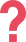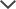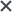My daughter used the NNAT practice tests. Her results after 4 weeks: 133; "very superior". The highest score ranking percentile she could get was a 99, she got a 98. We could not ask for more!
José
Thank you for your superb customer service; and thank you for making a difference in my life. I am happy to let you know that I passed the test.
Helen
I got the ACCUPLACER pack. I found TestPrep-Online’s preparation pack very helpful. The questions and study guides are great. I found that the explanations and solving tips really helped me to learn the material and improve my score.
Nicole
I used the LSAT practice material. It helped improve my technique a lot.
Steve
I got the TEAS tests. I found this website to be very helpful. The practice tests are almost identical to the real test; they have helped me a lot in studying.
Chelsea
My son could practice the types of questions and have an understanding of what he was going to be asked and confidence going into the test.
Christopher

## What is the ACCUPLACER Elementary Algebra Test?

The ACCUPLACER Elementary Algebra exam evaluates your ability to solve problems that involve elementary algebraic concepts and basic algebraic operations. In total, there are 12 elementary algebra questions. The ACCUPLACER Elementary Algebra test is one of three tests that encompass the ACCUPLACER math placement test.

## ACCUPLACER Elementary Algebra Practice Tests

Prepare for the ACCUPLACER Elementary Algebra exam along with the other two ACCUPLACER test topics; the ACCUPLACER Arithmetic and ACCUPLACER College-Level Math tests. Our practice tests provide hundreds of questions accompanied by answers and explanations to assist your learning.

Discover new methods to answer questions and test your knowledge with our ACCUPLACER math practice tests.

## ACCUPLACER Elementary Algebra Test Topics

The ACCUPLACER math placement test evaluates your knowledge in three topics, listed below:

### Integers and rational numbers

• Computing with integers and rational numbers
• Absolute values
• Number ordering

### Algebraic expressions

• Evaluating formulas and algebraic expressions
• Arithmetic operations with algebraic fractions
• Basic arithmetic involving monomials and polynomials
• Evaluating positive rational roots and exponents
• Factoring

### Equations, inequalities, and verbal problems

• Verbal algebraic problems including geometric reasoning
• Graphing

Master these topics and get a high score on your ACCUPLACER Math Elementary Algebra exam. Prepare for the math placement test with our abundant ACCUPLACER resources. We offer ACCUPLACER practice tests with questions that cover the same material found in all three math sections. In addition, you will find comprehensive practice for the English questions. Start improving your score now.

## Elementary Algebra Sample Questions for ACCUPLACER

Start preparing for the ACCUPLACER Elementary Algebra placement exam using our free questions below. Each question is accompanied by an answer and explanation.

Question 1: Level 1 ACCUPLACER Elementary Algebra Question

Calculate the value of x:1. 0
2. 1.5
3. 3
4. 4

Scroll down to reveal the answer and explanation.

To solve this problem, apply the equation rules of operations. Simplify the equation by reducing and moving like-terms:According to the calculations, the correct answer is (A). If you chose any other answer, review your calculations.

Question 2: Level 2 ACCUPLACER Elementary Algebra Question

Every day that Jim eats oatmeal for breakfast, his father gives him 10 smiley stickers. Every day that Jim eats something other than oatmeal for breakfast, he gives back 5 smiley stickers. The number of days that Jim ate oatmeal for breakfast this year is represented by c, and the number of days he ate something other than oatmeal for breakfast this year is represented by d. Which of the following represents the number of stickers Jim holds at the end of the year?

1.1.1.1.Scroll down to reveal the answer and explanation.

Write down all the information given in the question.

The number of days Jim ate oatmeal for breakfast this year: c

The number of days Jim ate something other than oatmeal this year: d

The number of stickers given to Jim by his father: 10

The number of stickers Jim is left with after giving to his father: −5

The total number of stickers Jim has at the end of the year is 10c−5d; therefore, the correct answer is (B).

If you chose any other answer, review the setup of your equation.

Question 3: Level 3 ACCUPLACER Elementary Algebra Question

Which of the following is equivalent to:1. 1

1.1.1.Scroll down to reveal the answer and explanation.

To simplify the expression, the numerator and the denominator should be factored independently.

Finding the factored form of the numerator:is a quadratic expression with the coefficients:The roots of this quadratic expression are expressed by:Hence:ACCUPLACER Math Elementary Algebra Practice Question is equivalent to.

As part of the quadratic expression definition, the equation equals zero; thus, you can manipulate it:Rewrite the equation:Therefore, the correct answer is D.

If you chose any other answer, review your calculations and the rules of factoring.

## ACCUPLACER Math Study Guide – Free Algebra Questions

Try free elementary algebra questions for ACCUPLACER. Download our free ACCUPLACER PDF with 30 questions that cover both the math and English sections of the ACCUPLACER.
Get answer explanations for each question and begin your preparation for the ACCUPLACER college placement test.Get Your Free ACCUPLACER Study Guide PDF Here

## Begin Your ACCUPLACER Elementary Algebra Practice Now

Start college off on the right foot. If you have a college math placement test coming up, arrive prepared and get the score you need. Prepare for the elementary algebra section on the ACCUPLACER with TestPrep-Online’s practice packs.

For math-only practice, get the ACCUPLACER Math Pack. For ACCUPLACER English prep and Math prep combined, go for the comprehensive ACCUPLACER Premium Pack. Improve your performance, increase your confidence, and avoid unnecessary remedial classes!

ACCUPLACER® and WritePlacer® are trademarks and property of COLLEGE BOARD. The trademark holder is not affiliated with TestPrep-Online or this website.

Need HelpNeed Help